Principles of Accounting, Volume 1: Financial Accounting

# 11.3Explain and Apply Depreciation Methods to Allocate Capitalized Costs

Principles of Accounting, Volume 1: Financial Accounting11.3 Explain and Apply Depreciation Methods to Allocate Capitalized Costs

In this section, we concentrate on the major characteristics of determining capitalized costs and some of the options for allocating these costs on an annual basis using the depreciation process. In the determination of capitalized costs, we do not consider just the initial cost of the asset; instead, we determine all of the costs necessary to place the asset into service. For example, if our company purchased a drill press for $22,000, and spent$2,500 on sales taxes and $800 for delivery and setup, the depreciation calculation would be based on a cost of$22,000 plus $2,500 plus$800, for a total cost of $25,300. We also address some of the terminology used in depreciation determination that you want to familiarize yourself with. Finally, in terms of allocating the costs, there are alternatives that are available to the company. We consider three of the most popular options, the straight-line method, the units-of-production method, and the double-declining-balance method. ### Your Turn #### Calculating Depreciation Costs Liam buys his silk screen machine for$10,000. He estimates that he can use this machine for five years or 100,000 presses, and that the machine will only be worth $1,000 at the end of its life. He also estimates that he will make 20,000 clothing items in year one and 30,000 clothing items in year two. Determine Liam’s depreciation costs for his first two years of business under straight-line, units-of-production, and double-declining-balance methods. Also, record the journal entries. Solution Straight-line method: ($10,000 – $1,000)/5 =$1,800 per year for both years.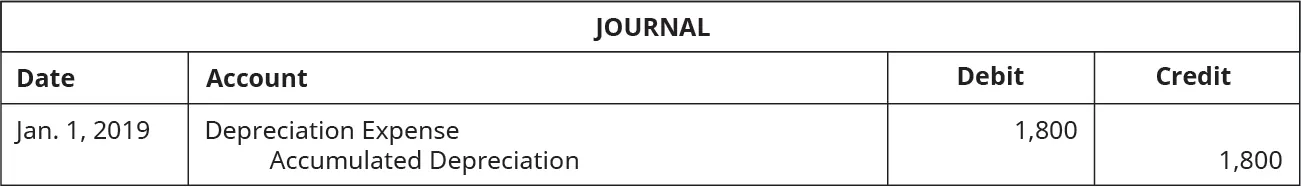Units-of-production method: ($10,000 –$1,000)/100,000= $0.09 per press Year 1 expense:$0.09 × 20,000 = $1,800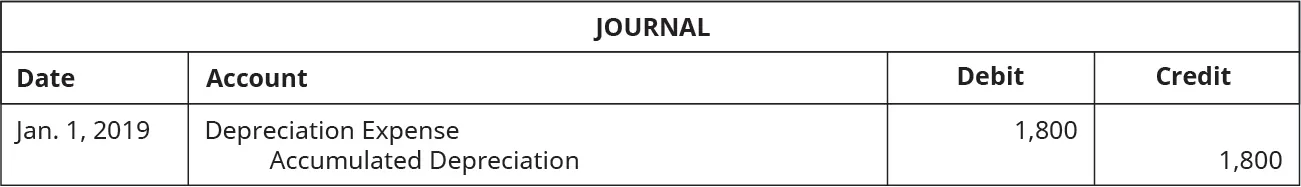Year 2 expense:$0.09 × 30,000 = $2,700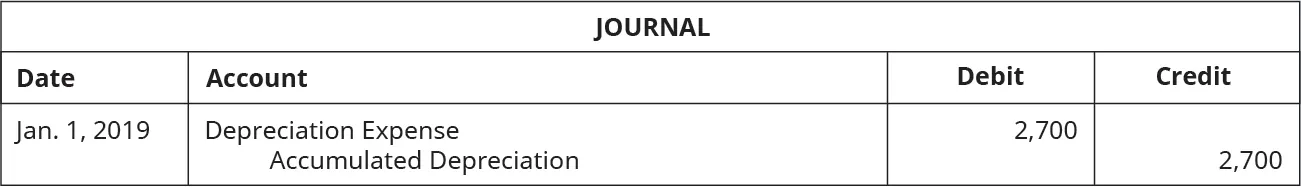Double-declining-balance method: Year 1 expense: [($10,000 – 0)/5] × 2 = $4,000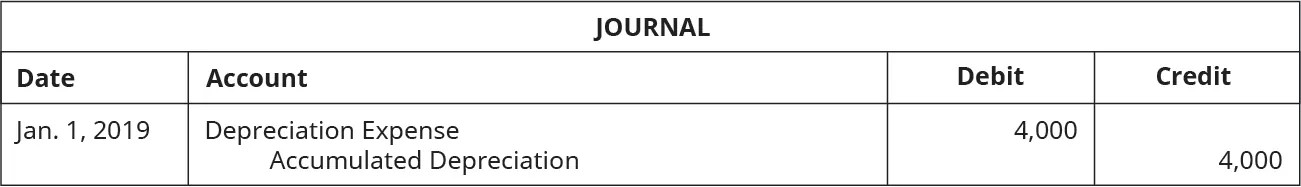Year 2 expense: [($10,000 – $4,000)/5] × 2 =$2,400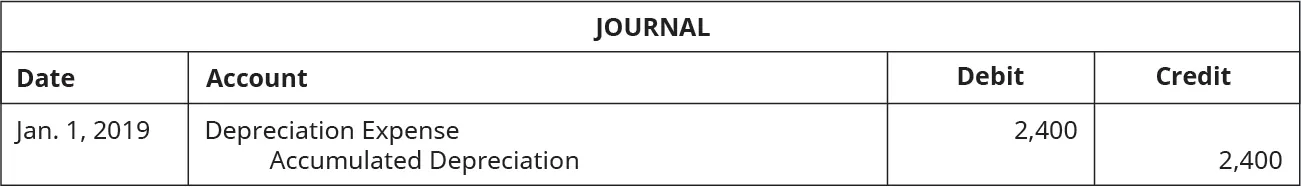### Fundamentals of Depreciation

As you have learned, when accounting for a long-term fixed asset, we cannot simply record an expense for the cost of the asset and record the entire outflow of cash in one accounting period. Like all other assets, when purchasing or acquiring a long-term asset, it must be recorded at the historical (initial) cost, which includes all costs to acquire the asset and put it into use. The initial recording of an asset has two steps:

1. Record the initial purchase on the date of purchase, which places the asset on the balance sheet (as property, plant, and equipment) at cost, and record the amount as notes payable, accounts payable, or an outflow of cash.
2. At the end of the period, make an adjusting entry to recognize the depreciation expense. Companies may record depreciation expense incurred annually, quarterly, or monthly.

Following GAAP and the expense recognition principle, the depreciation expense is recognized over the asset’s estimated useful life.

#### Recording the Initial Purchase of an Asset

Assets are recorded on the balance sheet at cost, meaning that all costs to purchase the asset and to prepare the asset for operation should be included. Costs outside of the purchase price may include shipping, taxes, installation, and modifications to the asset.

The journal entry to record the purchase of a fixed asset (assuming that a note payable is used for financing and not a short-term account payable) is shown here.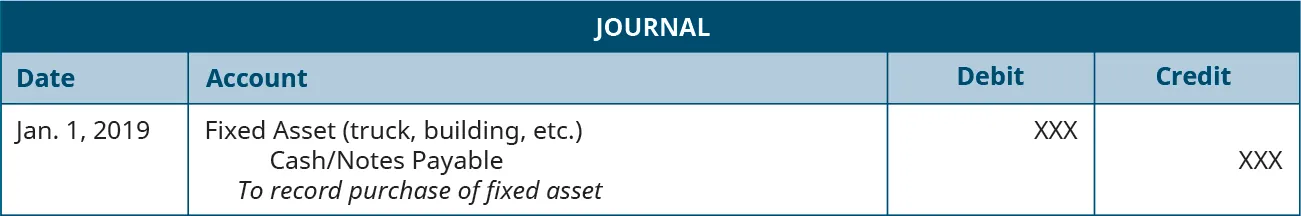Applying this to Liam’s silk-screening business, we learn that he purchased his silk-screening machine for $5,000 by paying$1,000 cash and the remainder in a note payable over five years. The journal entry to record the purchase is shown here.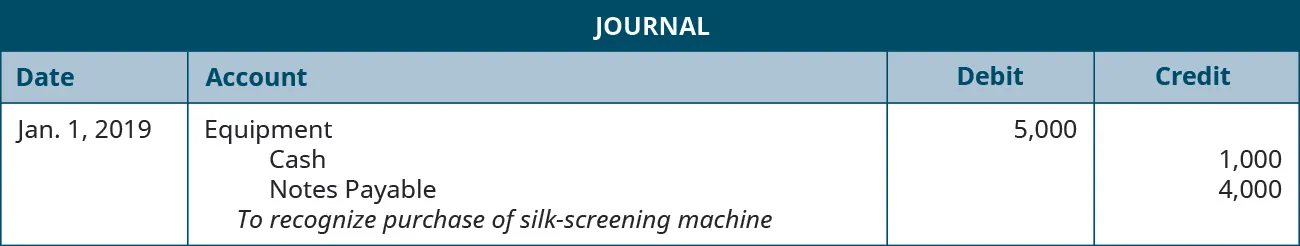### Concepts In Practice

Useful life and salvage value are estimates made at the time an asset is placed in service. It is common and expected that the estimates are inaccurate with the uncertainty involved in estimating the future. Sometimes, however, a company may attempt to take advantage of estimating salvage value and useful life to improve earnings. A larger salvage value and longer useful life decrease annual depreciation expense and increase annual net income. An example of this behavior is Waste Management, which was disciplined by the Securities and Exchange Commission for fraudulently altering its estimates to reduce depreciation expense and overstate net income by $1.7 billion.6 #### Components Used in Calculating Depreciation The expense recognition principle that requires that the cost of the asset be allocated over the asset’s useful life is the process of depreciation. For example, if we buy a delivery truck to use for the next five years, we would allocate the cost and record depreciation expense across the entire five-year period. The calculation of the depreciation expense for a period is not based on anticipated changes in the fair market value of the asset; instead, the depreciation is based on the allocation of the cost of owning the asset over the period of its useful life. The following items are important in determining and recording depreciation: • Book value: the asset’s original cost less accumulated depreciation. • Useful life: the length of time the asset will be productively used within operations. • Salvage (residual) value: the price the asset will sell for or be worth as a trade-in when its useful life expires. The determination of salvage value can be an inexact science, since it requires anticipating what will occur in the future. Often, the salvage value is estimated based on past experiences with similar assets. • Depreciable base (cost): the depreciation expense over the asset’s useful life. For example, if we paid$50,000 for an asset and anticipate a salvage value of $10,000, the depreciable base is$40,000. We expect $40,000 in depreciation over the time period in which the asset was used, and then it would be sold for$10,000.

Depreciation records an expense for the value of an asset consumed and removes that portion of the asset from the balance sheet. The journal entry to record depreciation is shown here.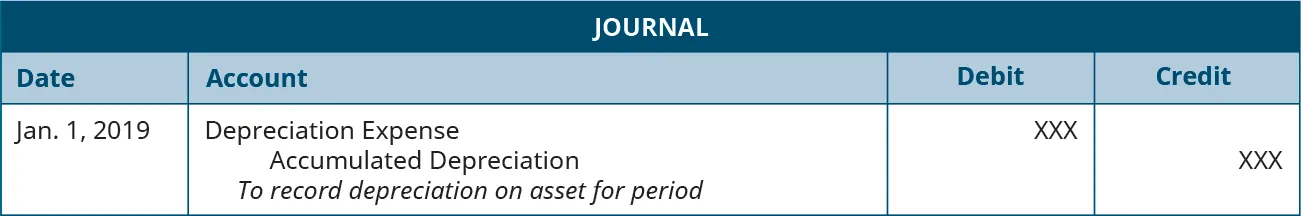Depreciation expense is a common operating expense that appears on an income statement. Accumulated depreciation is a contra account, meaning it is attached to another account and is used to offset the main account balance that records the total depreciation expense for a fixed asset over its life. In this case, the asset account stays recorded at the historical value but is offset on the balance sheet by accumulated depreciation. Accumulated depreciation is subtracted from the historical cost of the asset on the balance sheet to show the asset at book value. Book value is the amount of the asset that has not been allocated to expense through depreciation.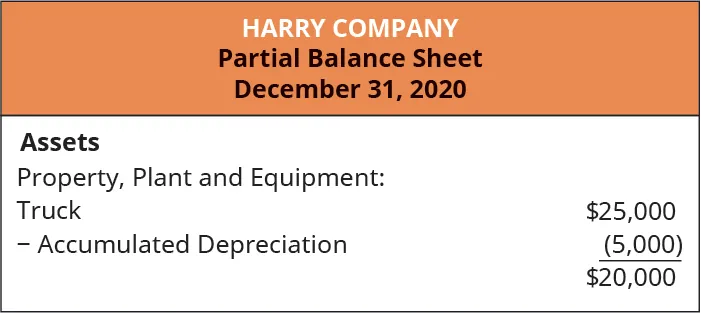In this case, the asset’s book value is $20,000: the historical cost of$25,000 less the accumulated depreciation of $5,000. It is important to note, however, that not all long-term assets are depreciated. For example, land is not depreciated because depreciation is the allocating of the expense of an asset over its useful life. How can one determine a useful life for land? It is assumed that land has an unlimited useful life; therefore, it is not depreciated, and it remains on the books at historical cost. Once it is determined that depreciation should be accounted for, there are three methods that are most commonly used to calculate the allocation of depreciation expense: the straight-line method, the units-of-production method, and the double-declining-balance method. A fourth method, the sum-of-the-years-digits method, is another accelerated option that has been losing popularity and can be learned in intermediate accounting courses. Let’s use the following scenario involving Kenzie Company to work through these three methods. Assume that on January 1, 2019, Kenzie Company bought a printing press for$54,000. Kenzie pays shipping costs of $1,500 and setup costs of$2,500, assumes a useful life of five years or 960,000 pages. Based on experience, Kenzie Company anticipates a salvage value of $10,000. Recall that determination of the costs to be depreciated requires including all costs that prepare the asset for use by the company. The Kenzie example would include shipping and setup costs. Any costs for maintaining or repairing the equipment would be treated as regular expenses, so the total cost would be$58,000, and, after allowing for an anticipated salvage value of $10,000 in five years, the business could take$48,000 in depreciation over the machine’s economic life.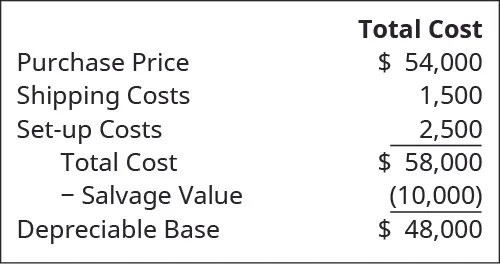### Concepts In Practice

#### Fixed Assets

You work for Georgia-Pacific as an accountant in charge of the fixed assets subsidiary ledger at a production and warehouse facility in Pennsylvania. The facility is in the process of updating and replacing several asset categories, including warehouse storage units, fork trucks, and equipment on the production line. It is your job to keep the information in the fixed assets subsidiary ledger up to date and accurate. You need information on original historical cost, estimated useful life, salvage value, depreciation methods, and additional capital expenditures. You are excited about the new purchases and upgrades to the facility and how they will help the company serve its customers better. However, you have been in your current position for only a few years and have never overseen extensive updates, and you realize that you will have to gather a lot of information at once to keep the accounting records accurate. You feel overwhelmed and take a minute to catch your breath and think through what you need. After a few minutes, you realize that you have many people and many resources to work with to tackle this project. Whom will you work with and how will you go about gathering what you need?

### Straight-Line Depreciation

Straight-line depreciation is a method of depreciation that evenly splits the depreciable amount across the useful life of the asset. Therefore, we must determine the yearly depreciation expense by dividing the depreciable base of $48,000 by the economic life of five years, giving an annual depreciation expense of$9,600. The journal entries to record the first two years of expenses are shown, along with the balance sheet information. Here are the journal entry and information for year one: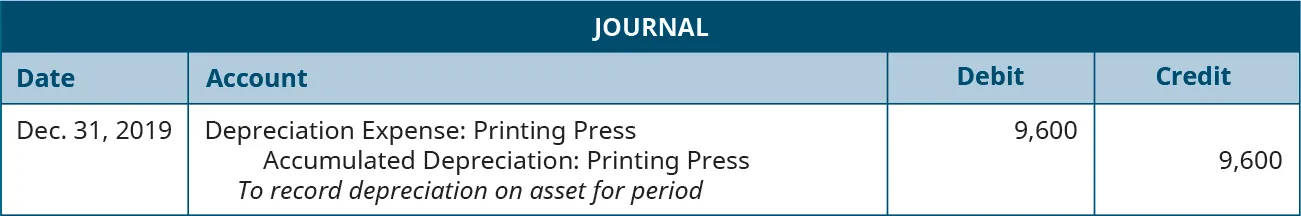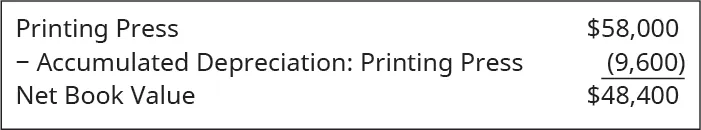After the journal entry in year one, the press would have a book value of $48,400. This is the original cost of$58,000 less the accumulated depreciation of $9,600. Here are the journal entry and information for year two: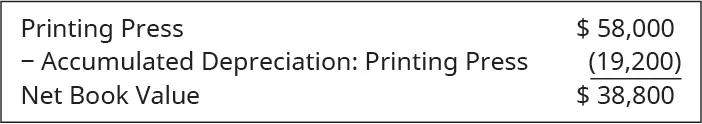Kenzie records an annual depreciation expense of$9,600. Each year, the accumulated depreciation balance increases by $9,600, and the press’s book value decreases by the same$9,600. At the end of five years, the asset will have a book value of $10,000, which is calculated by subtracting the accumulated depreciation of$48,000 (5 × $9,600) from the cost of$58,000.

### Units-of-Production Depreciation

The net effect of the differences in straight-line depreciation versus double-declining-balance depreciation is that under the double-declining-balance method, the allowable depreciation expenses are greater in the earlier years than those allowed for straight-line depreciation. However, over the depreciable life of the asset, the total depreciation expense taken will be the same, no matter which method the entity chooses. For example, in the current example both straight-line and double-declining-balance depreciation will provide a total depreciation expense of $48,000 over its five-year depreciable life. ### IFRS Connection #### Accounting for Depreciation Both US GAAP and International Financial Reporting Standards (IFRS) account for long-term assets (tangible and intangible) by recording the asset at the cost necessary to make the asset ready for its intended use. Additionally, both sets of standards require that the cost of the asset be recognized over the economic, useful, or legal life of the asset through an allocation process such as depreciation. However, there are some significant differences in how the allocation process is used as well as how the assets are carried on the balance sheet. IFRS and US GAAP allow companies to choose between different methods of depreciation, such as even allocation (straight-line method), depreciation based on usage (production methods), or an accelerated method (double-declining balance). The mechanics of applying these methods do not differ between the two standards. However, IFRS requires companies to use “component depreciation” if it is feasible. Component depreciation would apply to assets with components that have differing lives. Consider the following example using a plane owned by Southwest Airlines. Let’s divide this plane into three components: the interior, the engines, and the fuselage. Suppose the average life of the interior of a plane is ten years, the average life of the engines is fifteen years, and the average life of the fuselage is twenty-five years. Given this, what should be the depreciable life of the asset? In that case, under IFRS, the costs associated with the interior would be depreciated over ten years, the costs associated with the engines would be depreciated over fifteen years, and the costs associated with the fuselage would be depreciated over twenty-five years. Under US GAAP, the total cost of the airplane would likely be depreciated over twenty years. Obviously, component depreciation involves more record keeping and differing amounts of depreciation per year for the life of the asset. But the same amount of total depreciation, the cost of the asset less residual value, would be taken over the life of the asset under both US GAAP and IFRS. Probably one of the most significant differences between IFRS and US GAAP affects long-lived assets. This is the ability, under IFRS, to adjust the value of those assets to their fair value as of the balance sheet date. The adjustment to fair value is to be done by “class” of asset, such as real estate, for example. A company can adjust some classes of assets to fair value but not others. Under US GAAP, almost all long-lived assets are carried on the balance sheet at their depreciated historical cost, regardless of how the actual fair value of the asset changes. Consider the following example. Suppose your company owns a single building that you bought for$1,000,000. That building currently has $200,000 in accumulated depreciation. This building now has a book value of$800,000. Under US GAAP, this is how this building would appear in the balance sheet. Even if the fair value of the building is $875,000, the building would still appear on the balance sheet at its depreciated historical cost of$800,000 under US GAAP. Alternatively, if the company used IFRS and elected to carry real estate on the balance sheet at fair value, the building would appear on the company’s balance sheet at its new fair value of $875,000. It is difficult to determine an accurate fair value for long-lived assets. This is one reason US GAAP has not permitted the fair valuing of long-lived assets. Different appraisals can result in different determinations of “fair value.” Thus, the Financial Accounting Standards Board (FASB) elected to continue with the current method of carrying assets at their depreciated historical cost. The thought process behind the adjustments to fair value under IFRS is that fair value more accurately represents true value. Even if the fair value reported is not known with certainty, reporting the class of assets at a reasonable representation of fair value enhances decision-making by users of the financial statements. ### Summary of Depreciation Table 11.2 compares the three methods discussed. Note that although each time-based (straight-line and double-declining balance) annual depreciation expense is different, after five years the total amount depreciated (accumulated depreciation) is the same. This occurs because at the end of the asset’s useful life, it was expected to be worth$10,000: thus, both methods depreciated the asset’s value by $48,000 over that time period. The units of production method is different from the two above methods in that while those methods are based on time factors, the units of production is based on usage. However, the total amount of depreciation taken over an asset’s economic life will still be the same. In our example, the total depreciation will be$48,000, even though the sum-of-the-years-digits method could take only two or three years or possibly six or seven years to be allocated.

Calculation of Depreciation Expense
Depreciation Method Calculation
Straight line (Cost – salvage value)/Useful life
Units of production (Cost – salvage value) × (Units produced in current period/Estimated total units to be produced)
Double declining balance Book value × Straight-line annual depreciation percentage × 2
Table 11.2### Ethical Considerations

#### Depreciation Analysis Requires Careful Evaluation

When analyzing depreciation, accountants are required to make a supportable estimate of an asset’s useful life and its salvage value. However, “management teams typically fail to invest either time or attention into making or periodically revisiting and revising reasonably supportable estimates of asset lives or salvage values, or the selection of depreciation methods, as prescribed by GAAP.”7 This failure is not an ethical approach to properly accounting for the use of assets.

Accountants need to analyze depreciation of an asset over the entire useful life of the asset. As an asset supports the cash flow of the organization, expensing its cost needs to be allocated, not just recorded as an arbitrary calculation. An asset’s depreciation may change over its life according to its use. If asset depreciation is arbitrarily determined, the recorded “gains or losses on the disposition of depreciable property assets seen in financial statements”8 are not true best estimates. Due to operational changes, the depreciation expense needs to be periodically reevaluated and adjusted.

Any mischaracterization of asset usage is not proper GAAP and is not proper accrual accounting. Therefore, “financial statement preparers, as well as their accountants and auditors, should pay more attention to the quality of depreciation-related estimates and their possible mischaracterization and losses of credits and charges to operations as disposal gains.”9 An accountant should always follow GAAP guidelines and allocate the expense of an asset according to its usage.

### Partial-Year Depreciation

A company will usually only own depreciable assets for a portion of a year in the year of purchase or disposal. Companies must be consistent in how they record depreciation for assets owned for a partial year. A common method is to allocate depreciation expense based on the number of months the asset is owned in a year. For example, a company purchases an asset with a total cost of $58,000, a five-year useful life, and a salvage value of$10,000. The annual depreciation is $9,600 ([$58,000 – 10,000]/5). However, the asset is purchased at the beginning of the fourth month of the fiscal year. The company will own the asset for nine months of the first year. The depreciation expense of the first year is $7,200 ($9,600 × 9/12). The company will depreciate the asset $9,600 for the next four years, but only$2,400 in the sixth year so that the total depreciation of the asset over its useful life is the depreciable amount of $48,000 ($7,200 + 9,600 + 9,600 + 9,600 + 9,600 + 2,400).

You are part of a team reviewing the financial statements of a new computer company. Looking over the fixed assets accounts, one long-term tangible asset sticks out. It is labeled “USB” and valued at $10,000. You ask the company’s accountant for more detail, and he explains that the asset is a USB drive that holds the original coding for a game the company developed during the year. The company expects the game to be fairly popular for the next few years, and then sales are expected to trail off. Because of this, they are planning on depreciating this asset over the next five years using the double-declining method. Does this recording seem appropriate, or is there a better way to categorize the asset? How should this asset be expensed over time? ### Special Issues in Depreciation While you’ve now learned the basic foundation of the major available depreciation methods, there are a few special issues. Until now, we have assumed a definite physical or economically functional useful life for the depreciable assets. However, in some situations, depreciable assets can be used beyond their useful life. If so desired, the company could continue to use the asset beyond the original estimated economic life. In this case, a new remaining depreciation expense would be calculated based on the remaining depreciable base and estimated remaining economic life. Assume in the earlier Kenzie example that after five years and$48,000 in accumulated depreciation, the company estimated that it could use the asset for two more years, at which point the salvage value would be $0. The company would be able to take an additional$10,000 in depreciation over the extended two-year period, or $5,000 a year, using the straight-line method. As with the straight-line example, the asset could be used for more than five years, with depreciation recalculated at the end of year five using the double-declining balance method. While the process of calculating the additional depreciation for the double-declining-balance method would differ from that of the straight-line method, it would also allow the company to take an additional$10,000 after year five, as with the other methods, so long as the cost of $58,000 is not exceeded. As a side note, there often is a difference in useful lives for assets when following GAAP versus the guidelines for depreciation under federal tax law, as enforced by the Internal Revenue Service (IRS). This difference is not unexpected when you consider that tax law is typically determined by the United States Congress, and there often is an economic reason for tax policy. For example, if we want to increase investment in real estate, shortening the economic lives of real estate for taxation calculations can have a positive increasing effect on new construction. If we want to slow down new production, extending the economic life can have the desired slowing effect. In this course, we concentrate on financial accounting depreciation principles rather than tax depreciation. ### Fundamentals of Depletion of Natural Resources Another type of fixed asset is natural resources, assets a company owns that are consumed when used. Examples include lumber, mineral deposits, and oil/gas fields. These assets are considered natural resources while they are still part of the land; as they are extracted from the land and converted into products, they are then accounted for as inventory (raw materials). Natural resources are recorded on the company’s books like a fixed asset, at cost, with total costs including all expenses to acquire and prepare the resource for its intended use. As the resource is consumed (converted to a product), the cost of the asset must be expensed: this process is called depletion. As with depreciation of nonnatural resource assets, a contra account called accumulated depletion, which records the total depletion expense for a natural resource over its life, offsets the natural resource asset account. Depletion expense is typically calculated based on the number of units extracted from cutting, mining, or pumping the resource from the land, similar to the units-of-production method. For example, assume a company has an oil well with an estimated 10,000 gallons of crude oil. The company purchased this well for$1,000,000, and the well is expected to have no salvage value once it is pumped dry. The depletion cost per gallon will be $1,000,000/10,000 =$100. If the company extracts 4,000 gallons of oil in a given year, the depletion expense will be $400,000. ### Fundamentals of Amortization of an Intangible Recall that intangible assets are recorded as long-term assets at their cost. As with tangible assets, many intangible assets have a finite (limited) life span so their costs must be allocated over their useful lives: this process is amortization. Depreciation and amortization are similar in nature but have some important differences. First, amortization is typically only done using the straight-line method. Second, there is usually no salvage value for intangible assets because they are completely used up over their life span. Finally, an accumulated amortization account is not required to record yearly expenses (as is needed with depreciation); instead, the intangible asset account is written down each period. For example, a company called Patents-R-Us purchased a product patent for$10,000, granting the company exclusive use of that product for the next twenty years. Therefore, unless the company does not think the product will be useful for all twenty years (at that point the company would use the shorter useful life of the product), the company will record amortization expense of $500 a year ($10,000/20 years). Assuming that it was placed into service on October 1, 2019, the journal entry would be as follows: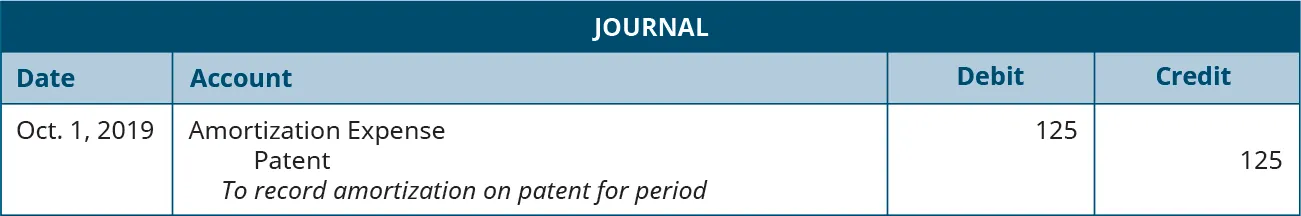### Footnotes

• 6U.S. Securities and Exchange Commission. “Judge Enters Final Judgment against Former CFO of Waste Management, Inc. Following Jury Verdict in SEC’s Favor.” January 3, 2008. https://www.sec.gov/news/press/2008/2008-2.htm
• 7Howard B. Levy. “Depreciable Asset Lives.” The CPA Journal. September 2016. https://www.cpajournal.com/2016/09/08/depreciable-asset-lives/
• 8Howard B. Levy. “Depreciable Asset Lives.” The CPA Journal. September 2016. https://www.cpajournal.com/2016/09/08/depreciable-asset-lives/
• 9Howard B. Levy. “Depreciable Asset Lives.” The CPA Journal. September 2016. https://www.cpajournal.com/2016/09/08/depreciable-asset-lives/
Order a print copy

As an Amazon Associate we earn from qualifying purchases.